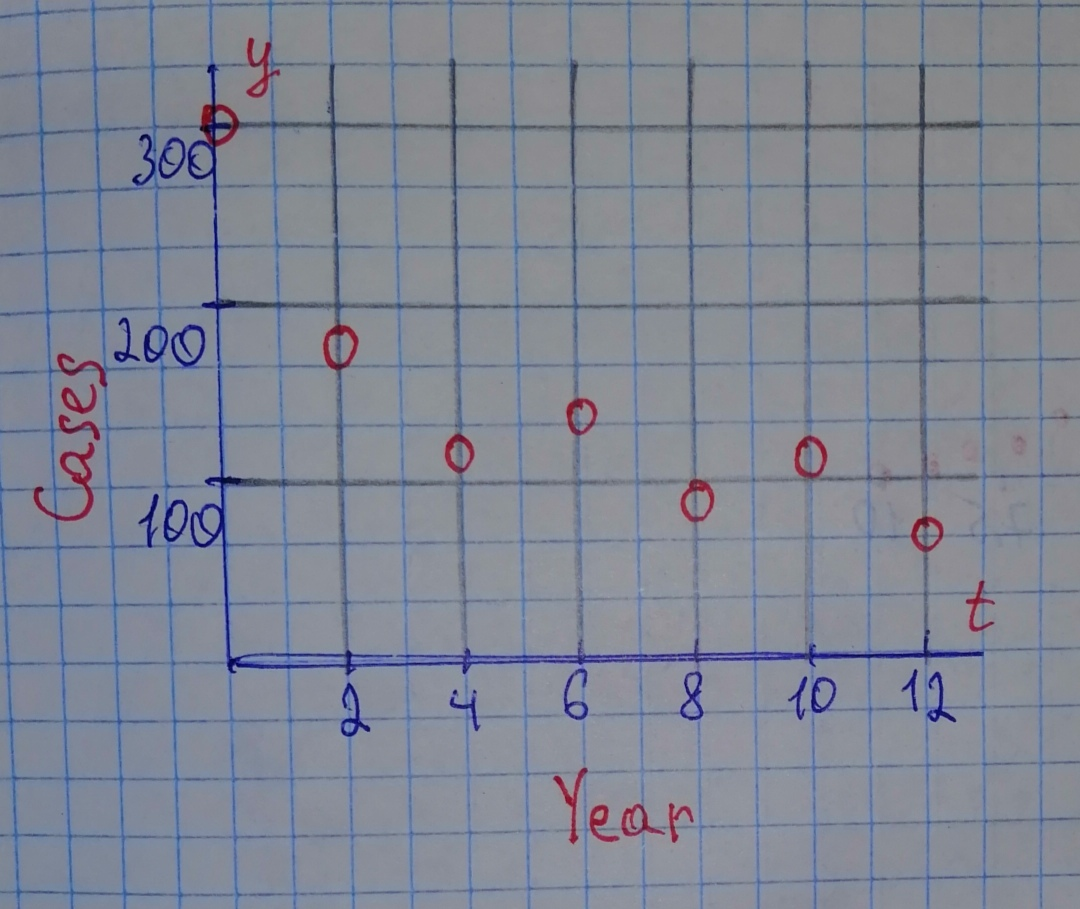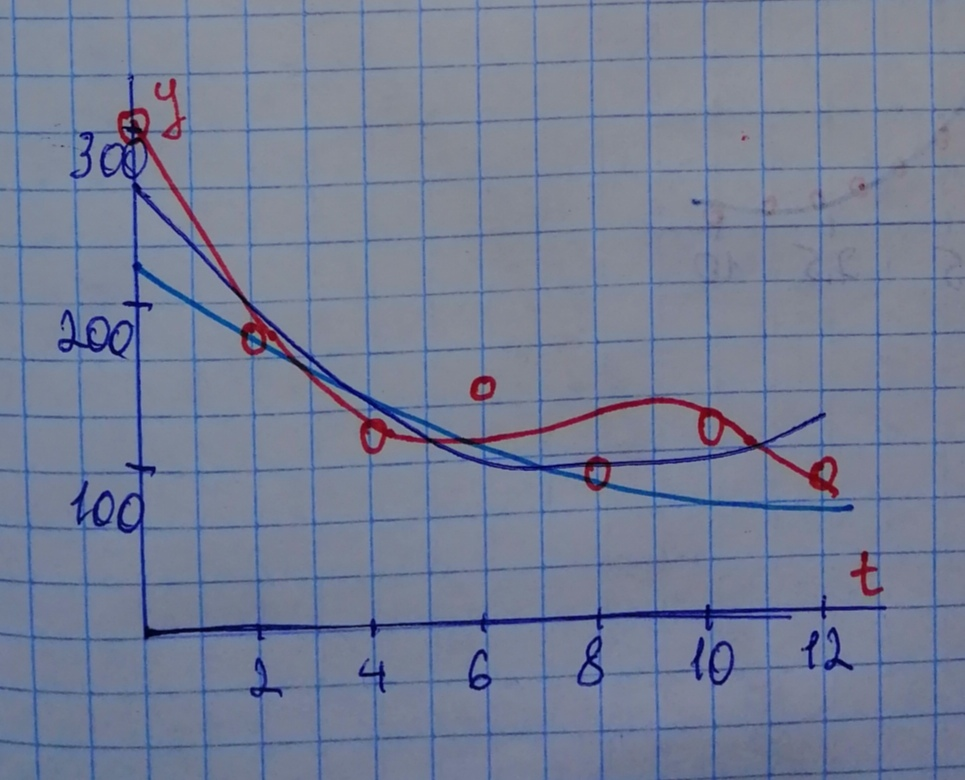#### Didn’t find what you are looking for?

Question# The following table lists the reported number of cases of infants born in the United States with HIV in recent years because their mother was infected. Source:Centers for Disease Control

Exponential models
ANSWEREDThe following table lists the reported number of cases of infants born in the United States with HIV in recent years because their mother was infected.
Source:
Centers for Disease Control and Prevention.
$$\begin{array}{|c|c|}\hline \text{Year} & \text{Cases} \\ \hline 1995 & 295 \\ \hline 1997 & 166 \\ \hline 1999 & 109 \\ \hline 2001 & 115 \\ \hline 2003 & 94 \\ \hline 2005 & 107 \\ \hline 2007 & 79 \\ \hline \end{array}$$
a) Plot the data on a graphing calculator, letting $$\displaystyle{t}={0}$$ correspond to the year 1995.
b) Using the regression feature on your calculator, find a quadratic, a cubic, and an exponential function that models this data.
c) Plot the three functions with the data on the same coordinate axes. Which function or functions best capture the behavior of the data over the years plotted?
d) Find the number of cases predicted by all three functions for 20152015. Which of these are realistic? Explain.2020-11-09

Step 1
Answer for (a): The following table lists the reported number of cases of infants born in the United States with HIV in recent years because their mother was infected
$$\begin{array}{|c|c|}\hline \text{Year} & \text{Cases} \\ \hline 1995 & 295 \\ \hline 1997 & 166 \\ \hline 1999 & 109 \\ \hline 2001 & 115 \\ \hline 2003 & 94 \\ \hline 2005 & 107 \\ \hline 2007 & 79 \\ \hline \end{array}$$
Now letting $$\displaystyle{t}={0}$$ corresponds to the year 1995 we get the following table.
$$\begin{array}{|c|c|}\hline \text{Year} & t & \text{Cases} \\ \hline 1995 & 0 & 295 \\ \hline 1997 & 2 & 166 \\ \hline 1999 & 4 & 109 \\ \hline 2001 & 6 & 115 \\ \hline 2003 & 8 & 94 \\ \hline 2005 & 10 & 107 \\ \hline 2007 & 12 & 79 \\ \hline \end{array}$$
Step 2
By using graphical calculator we have the following graph,Step 3
Answer for (b) Using the regression feature on our calculator, a quadratic, a cubic, and an exponential function that models the above data are given as
$$\displaystyle{y}={2.3839}{t}^{{{2}}}\ -\ {42.5536}{t}\ +\ {269.2143}$$
$$\displaystyle{y}=\ -{0.4931}{t}^{{{3}}}\ +\ {1.2589}{t}^{{{2}}}\ -\ {81.998}{t}\ +\ {292.881}$$
$$\displaystyle{y}={213.7921}{\left({0.9149}\right)}^{{{t}}}$$
Answer for(c) Let us plot above three function and data point on the same window we observe that the exponential function $$\displaystyle{y}={213.7921}{\left({0.9149}\right)}^{{{t}}}$$ is the best fit the data.Step 4
Answer for (d) $$\displaystyle{t}={20}$$ corresponds to the year 2015.
$$\displaystyle{y}{\left({20}\right)}={2.383}{\left({20}\right)}^{{{2}}}\ -\ {42.5536}{\left({20}\right)}\ +\ {269.2143}$$
$$\displaystyle={371.7023}\ \approx\ {372}$$
$$\displaystyle{y}{\left({20}\right)}=\ -{0.4931}{\left({20}\right)}^{{{3}}}\ +\ {11.2589}{\left({20}\right)}^{{{2}}}\ -\ {81.998}{\left({20}\right)}\ +\ {292.881}$$
$$\displaystyle=\ -{788.319}\ \approx\ -{788}$$
$$\displaystyle{y}{\left({20}\right)}={213.7921}{\left({0.9149}\right)}^{{{20}}}$$
$$\displaystyle={36.0964}\ \approx\ {36}$$
The only realistic value is given by the exponential function because the pattern of the data suggests that the number of cases decrease over time.